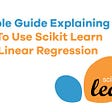# Basic concepts and mathematics

• The input or predictor variable is the variable(s) that help predict the value of the output variable. It is commonly referred to as X.
• The output variable is the variable that we want to predict. It is commonly referred to as Y.
1. It is used to predict the future values of continuous data.
2. Data Should be linear with a single independent and dependent variable.
`###Import necessary librariesimport numpy as npimport pandas as pdfrom matplotlib import pyplot as plt ### Generate 'random' datanp.random.seed(0)### Array of 100 values with mean = 1.5, stddev = 2.5x = 2.5 * np.random.randn(100) + 1.5 # Generate 100 residual terms  res = 0.5 * np.random.randn(100)# Actual values of YY = 2 + 0.3 * X + res                  # Create pandas dataframe to store our X and y valuesdf = pd.DataFrame( {'x': x,     'Y': Y}) # Show the first five rows of our dataframedf.head()`
`# Calculate the mean of X and yxmean = np.mean(x)ymean = np.mean(Y)# Calculate the terms needed for the numerator and denominator of betadf['xycov'] = (df['x'] - xmean) * (df['Y'] - ymean)df['xvar'] = (df['x'] - xmean)**2# Calculate beta and alphab1 = df['xycov'].sum() / df['xvar'].sum()b0 = ymean - (beta * xmean)print(f'b0 = {b0}')print(f'b1 = {b1}')`
`ypred = b0 + b1 x`
`# Plot regression against actual dataplt.figure(figsize=(12, 6))plt.plot(x, ypred)     # regression lineplt.plot(x, Y, 'ro')   # scatter plot showing actual dataplt.title('Actual vs Predicted')plt.xlabel('x')plt.ylabel('Y')plt.show()`
1. Multi Linear Regression is also used to predict the future values of continuous data.
2. Data Should be linear with multiple independent variables and a single dependent variable.
1. Polynomial Regression is used to predict the future values of continuous non-linear data.
2. Data Should be non-linear, with multiple independent variables and a single dependent variable.
1. Multi-variant Regression is used to predict multiple predictors with help of multiple response data.

“Don’t dream to join a tech giant company, dream to make a company gigantic.” — Sai

--

--

--

## More from sai krishna

A Machine Learning technology researcher commencing a new Blog Series to make clear ML concepts Simple and ease for everyone.

Love podcasts or audiobooks? Learn on the go with our new app.

## Dataism-At a glance## Fastest way to become a self-trained Data Scientist## Sports Analytics — Analyzing Cricket Dataset using MySQL## Have you heard of Zhua Zhou or “pick anniversary”?## Loan Data Prediction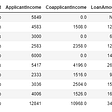## Time Series Analysis and Forecasting with Python## Learn Data Mining by Applying it on Excel(Part 2)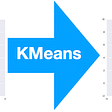## Insights from Seattle Airbnb data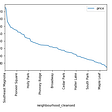## sai krishna

A Machine Learning technology researcher commencing a new Blog Series to make clear ML concepts Simple and ease for everyone.

## Machine Learning model for investing in companies of same Industry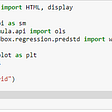## A simple guide explaining how to use scikit learn for linear regression